Courses

Test: Diode Circuits

15 Questions MCQ Test RRB JE for Electrical Engineering | Test: Diode Circuits

Description
Attempt Test: Diode Circuits | 15 questions in 45 minutes | Mock test for Electronics and Communication Engineering (ECE) preparation | Free important questions MCQ to study RRB JE for Electrical Engineering for Electronics and Communication Engineering (ECE) Exam | Download free PDF with solutions
QUESTION: 1

In the question a circuit and a waveform for the input voltage is given. The diode in circuit has cutin voltage Vγ = 0. Choose the option for the waveform of output voltage vo :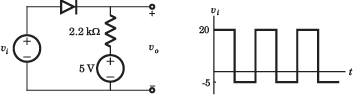​ ​ ​

Solution:
• Diode is off for vi  < 5 V. Hence vo = 5 V.
• For vi > 5 V, vo = vi .Therefore (D) is correct option.
QUESTION: 2

In the question a circuit and a waveform for the input voltage is given. The diode in circuit has cutin voltage Vγ = 0. Choose the option for the waveform of output voltage vo: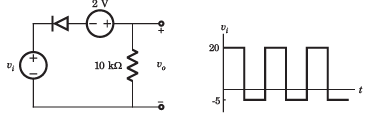Solution:
• Diode will be off if vi + 2 > 0.Thus vo = 0
• For v+ 2  < 0 V, vi  < -2, vo = vi + 2 = -3V
• Thus (C) is correct option
QUESTION: 3

In the question a circuit and a waveform for the input voltage is given. The diode in circuit has cutin voltage Vγ = 0. Choose the option for the waveform of output voltage vo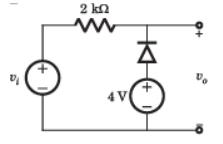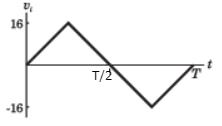Solution:
• For vi  < 4 V the diode is ON and output vo = 4 V.
• For vi  >4 V diode is off and output vo = vi.
• Thus (D) is correct option.
QUESTION: 4

In the question a circuit and a waveform for the input voltage is given. The diode in circuit has cutin voltage Vγ = 0. Choose the option for the waveform of output voltage vo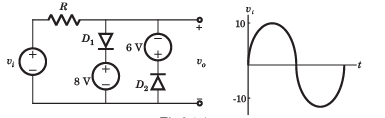Solution:
• During positive cycle when vs < 8V both diode are OFF vo = vs . For vs > 8V , vo = 8V , D1 is ON.
• During negative cycle when |vs| < 6 V, both diode are OFF, vo = vs .
• For |vs| > 6V , D2 is on v0 = -6V .Therefore (C) is correct.
QUESTION: 5

For the circuit in fig. P.3.1.5, let cutin voltage Vγ = 0.7 V. The plot of vo verses vi for -10 ≤ vi ≤10 V is: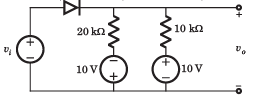Solution: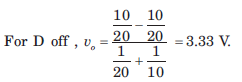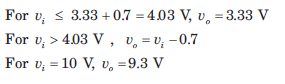QUESTION: 6

The circuit inside the box in fig. contains only resistor and diodes. The terminal voltage vo is connected to some point in the circuit inside the box. The largest and smallest possible value of vo most nearly to is respectively.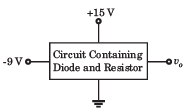Solution:

The output voltage cannot exceed the positive power supply voltage and cannot be lower than the negative power supply voltage.

QUESTION: 7

In the voltage regulator circuit in fig. the maximum load current iL that can be drawn is: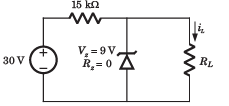Solution:

At regulated power supply: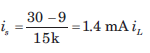will remain less than 1.4 mA.

QUESTION: 8

In the voltage regulator shown in fig. the power dissipation in the Zener diode is: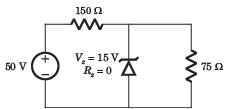Solution: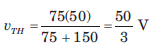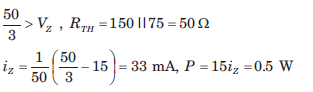QUESTION: 9

The Q-point for the Zener diode in fig. is: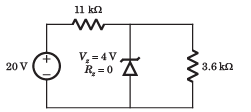Solution: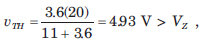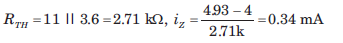QUESTION: 10

In the voltage regulator circuit in fig  the power rating of Zener diode is 400 mW. The value of RL that will establish maximum power in Zener diode is: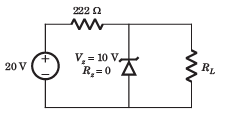Solution: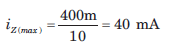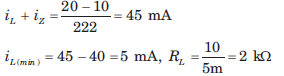QUESTION: 11

In the voltage regulator circuit in fig.  the Zener diode current is to be limited to the range 5 ≤ iz ≤100 mA.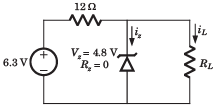The range of possible load current is:

Solution:

Current through 12 Ω resistor is: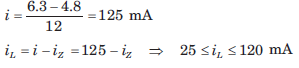QUESTION: 12

In the voltage regulator circuit in fig.  the Zener diode current is to be limited to the range 5 ≤ iz ≤100 mA.The range of possible load resistance is:

Solution: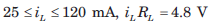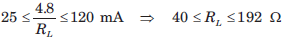QUESTION: 13

In the voltage regulator circuit in fig.  the Zener diode current is to be limited to the range 5 ≤ iz ≤100 mA.The power rating required for the load resistor is:

Solution: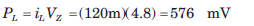QUESTION: 14

The secondary transformer voltage of the rectifier circuit shown in fig. is vs = 60 sin 2π 60tV. Each diode has a cut in voltage of Vγ = 0.6 V. The ripple voltage is to be no more than Vrip = 2 V. The value of filter capacitor will be: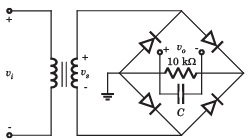Solution:

vs = 60 sin 2π 60tV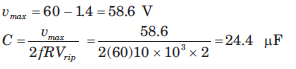QUESTION: 15

The input to full-wave rectifier in fig. is vi = 120 sin2π60t V. The diode cutin voltage is 0.7 V. If the output voltage cannot drop below 100 V, the required value of the capacitor is: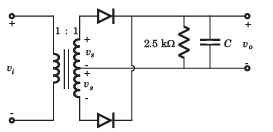Solution:

Full wave rectifier: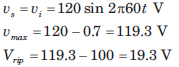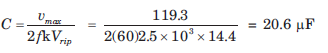Use Code STAYHOME200 and get INR 200 additional OFF Use Coupon Code

Track your progress, build streaks, highlight & save important lessons and more!

Similar ContentRelated tests